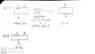# The potential difference/EMF in RL circuits

• hidemi
In summary, the inductance in a circuit will decay exponentially, reaching zero over time. The potential difference across the resistor will be close to zero.f

#### hidemi

Homework Statement
(1) An inductance L, resistance R, and ideal battery of emf ε are wired in series and the circuit is allowed to come to equilibrium. A switch in the circuit is opened at time t = 0, at which time the current is ε/R. At any later time t the potential difference across the resistor is given by:

A.ε (1−e^−Lt/R)
B.εe^−Lt/R
C.ε (1 +e^−Rt/L)
D.εe^−Rt/L
E. ε (1−e^−Rt/L) The answer is D.

(2) An inductance L, resistance R, and ideal battery of emf ε are wired in series. A switch in the circuit is closed at time t = 0, at which time the current is zero. At any later time t the emf of the inductor is given by:

A.ε (1−e^−Lt/R)
B.εe^−Lt/R
C.ε (1 +e^−Rt/L)
D.εe^−Rt/L
E. ε (1−e^−Rt/L) The answer is D.
Relevant Equations
Potential Difference = ε*e^(-Rt/L)
I would like to share my understanding of both of these questions.

For Question (1), the inductance L is releasing its stored energy to be dissipated by the resistance R. As time passes, the voltage across L is decreasing and thus the potential difference across the resistor will be close to 0.

For Question (2), the inductance L is being charged, as the current is given zero initially and thus voltage is at its maximum (because the inductance has a maximum reactance in the beginning). As time passes, the emf of the inductance drops (less reactance), so the emf of the inductor is close to 0.

Let me know if my thought is correct.

For (1), is there a diagram for the circuit in question?

If it is purely a series circuit and an inline switch is opened, where's the closed circuit to allow current to flow once that switch is opened?

•Steve4Physics
For (1), is there a diagram for the circuit in question?

If it is purely a series circuit and an inline switch is opened, where's the closed circuit to allow current to flow once that switch is opened?
There's no diagrams provided in both Question (1) and (2), but I could draw the diagrams from my own understanding. (See attachment)

#### Attachments

•1.jpg
27.7 KB · Views: 105
There's no diagrams provided in both Question (1) and (2), but I could draw the diagrams from my own understanding. (See attachment)
The descriptions in the Homework Statement aren’t quite correct. I think this is intended:
https://s3-us-west-2.amazonaws.com/...ites/222/2014/12/20110934/Figure_24_10_01.jpg
(from https://courses.lumenlearning.com/physics/chapter/23-10-rl-circuits/)

For Q1, switch is in position 1, circuit reaches equilibrium. At t=0 switch is changed to position 2.
For Q2, switch is in position 2, circuit reaches equilibrium. At t=0 switch is changed to position 1.

•hidemi
The descriptions in the Homework Statement aren’t quite correct. I think this is intended:
https://s3-us-west-2.amazonaws.com/...ites/222/2014/12/20110934/Figure_24_10_01.jpg
(from https://courses.lumenlearning.com/physics/chapter/23-10-rl-circuits/)

For Q1, switch is in position 1, circuit reaches equilibrium. At t=0 switch is changed to position 2.
For Q2, switch is in position 2, circuit reaches equilibrium. At t=0 switch is changed to position 1.
Thanks for the response!
I think your diagrams make more sense.
If those are the cases, then do my thoughts on post#1 still remain valid?

Thanks for the response!
I think your diagrams make more sense.
If those are the cases, then do my thoughts on post#1 still remain valid?
Your explanation for Q1 is OK but (being picky) rather than say
“the potential difference across the resistor will be close to 0.“
I would say something like
“the potential difference across the resistor decays exponentially (tending to zero for large times)”

For Q2 you said
“thus voltage is at its maximum (because the inductance has a maximum reactance in the beginning)”.
That's wrong.

You can’t use the term ‘reactance’ here because the concept of reactance is used for alternating current flowing through an inductor (inductive reactance ##X_L = 2\pi f L## wherre ##f## is the frequency).

I would say somethng like:
“thus emf is at its maximum (because the current is changing fastest at the beginning and induced emf is proportional to rate of change of current)”

•hidemi
Your explanation for Q1 is OK but (being picky) rather than say
“the potential difference across the resistor will be close to 0.“
I would say something like
“the potential difference across the resistor decays exponentially (tending to zero for large times)”

For Q2 you said
“thus voltage is at its maximum (because the inductance has a maximum reactance in the beginning)”.
That's wrong.

You can’t use the term ‘reactance’ here because the concept of reactance is used for alternating current flowing through an inductor (inductive reactance ##X_L = 2\pi f L## wherre ##f## is the frequency).

I would say somethng like:
“thus emf is at its maximum (because the current is changing fastest at the beginning and induced emf is proportional to rate of change of current)”